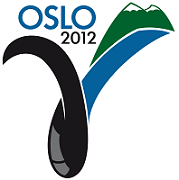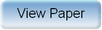Ninth International Geostatistics Congress, Oslo, Norway
June 11 – 15, 2012Session: Posters Abstract No.: P-019 Title: Use of Streamlines in Development and Application of a Linearized Reduced-Order Reservoir Model Author(s): M. Jesmani, Shiraz University (IR)B. Koohmare Hosseini, University of Alberta (CA)R.J. Chalaturnyk, University of Alberta (CA) Abstract: Reduced-order modeling procedures are very useful techniques where the simulation model must be run many times (i.e. optimization problems) or dimensions of the model are large. Therefore there is a significant need to reduce the computational requirements for the flow simulation model.This paper presents a new reduced-order linear model for a multi-phase flow in underground formations which is highly nonlinear. The proposed model takes advantage of streamlines using information gleaned from streamline trajectories. Mathematical methods (e.g. singular value decomposition) are frequently used in reservoir model order reduction procedures. In this work streamlines are used as an alternative to these classical techniques to decide which grid blocks? state variables are mostly effective on the model. Obviously the state variables are a complete description of a system. Therefore reservoir can be thoroughly described through state variables, related to the remaining streamlines of the cells.It is obvious that analyzing and controlling the behavior of a linear system is much easier than a nonlinear one. In this work, a novel linearization method in reservoir model is proposed. Dynamic variables of a reservoir such as pressure, have local dependency, which means their values depend only on neighboring grids? values and have no dependency in value to farther grids. This statement appears to be physically reasonable as well, since it abides by the reservoir fluid flow equations. Therefore dynamic variables of any curve can be estimated by dynamic variables of neighboring curves? grid blocks. In the present work, streamlines play the role of the so-called curves and the linear relationship between neighboring streamlines is developed employing least square method.It is shown that the model which is highly nonlinear in Cartesian or Radial coordinates, is linear in streamline based coordinates. The results of simulation on some synthetic cases verified the accuracy of the method.Produced by X-CD Technologies Inc.